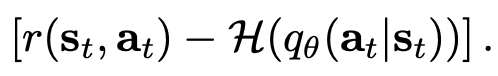# NaN issues with Reinforcement Learning in Pyro

I’m new to Pyro and I’m trying to implement a basic agent to solve the OpenAI gym cartpole exercise, using Sergey Levine’s (Reinforcement Learning and Control as Probabilistic Inference)[https://arxiv.org/pdf/1805.00909.pdf] tutorial. The basic structure is I sample a minibatch of trajectories (from the start until the cartpole falls over), then attempt to perform SVI on using this ELBO loss:where \$\$r(s_t, a_t)\$\$ is a reward given by the simulator, and the second term is the log probability of a given action conditioned on the state the agent is in.
First off, is there a good way to handle the dependence between the pyro.sample (for choosing the action) statements needed to create the trajectory/data? I’ve only seen examples like the VAE where the data is known ahead of time.
My second question is that right now I’m getting NaN issues on the first SVI step. Is there a good way to visualize the PyTorch computational graph to understand why I’m getting these issues?

I’ve attached my code, and I appreciate any pointers!

``````import math
import gym
import torch
from torch import nn
import pyro
import pyro.distributions as dist
from pyro.infer import SVI, Trace_ELBO, TraceGraph_ELBO, TraceEnum_ELBO

num_epochs = 2
steps_per_epoch = 5
num_rollouts = 1
rollout_length = 5
STATE_DIM = 4
ACTION_DIM = 2

class GuideDist(nn.Module):
"""
Basic linear perceptron with softmax, currently used
"""
def __init__(self):
super().__init__()
self.h1 = nn.Linear(STATE_DIM, ACTION_DIM)

def forward(self, state, timestep, batch_num):
pyro.module("posterior_guide", self)
action_probs = nn.functional.softmax(self.h1(state), dim=0)
#  DEBUG that softmax output is correct param for distribution
assert torch.logical_and(action_probs < 1,
0 < action_probs).all()
assert torch.isclose(torch.sum(action_probs),
torch.tensor(1.0))
pyro.sample("action_roll_{}_{}".format(batch_num, timestep),
dist.Categorical(action_probs))

def model(rollouts, lengths):
"""
Define the model for the agent
"""
# Assume a uniform action prior
action_probs = torch.ones(ACTION_DIM) / ACTION_DIM
#  for i in pyro.plate("rollouts", num_rollouts):
for i in range(num_rollouts):
rollout = rollouts[i]
length = lengths[i]
for t in range(length):
# We sample an action and observe the reward
pyro.sample("action_roll_{}_{}".format(i,t),
dist.Categorical(action_probs))
#  rollout[t,1] is the reward from rollout i at timestep t
pyro.sample("reward_roll_{}_t_{}".format(i,t),
dist.Uniform(math.exp(-1), 1), obs=rollout[t,1])

class CartpoleAgent:
#  How do we infer the policy_dist?
def __init__(self, max_timesteps_):
self.env = gym.make('CartPole-v1')
self.max_timesteps = max_timesteps_
self.policy = GuideDist()

def __del__(self):
self.env.close()

def sample_trajectories(self, num_rollouts):
# trajectory is list with [action, reward, observation] (observation
# being 4 elements
trajectories = torch.zeros(num_rollouts, self.max_timesteps,
6, dtype=torch.float32)
lengths = []
for i in range(num_rollouts):
self.env.reset()
#  Get initial state + initial reward is 1
state = self.env.reset()
for t in range(self.max_timesteps):
action = self.action(t, state, i)
next_state, reward, done, info = self.env.step(action)
#  Reward is always 1, thus e^(1-1) = 1 so leave it unchanged
trajectories[i,t] = torch.tensor([action, reward, *state],
dtype=torch.float32)
state = next_state
#  Take a step
if done:
break
lengths.append(t)
return trajectories, lengths

def render_trajectory(self):
observation = self.env.reset()
for t in range(self.max_timesteps):
self.env.render()
print(observation)
action = self.action(t, observation)
observation, reward, done, info = self.env.step(action)
if done:
print("Episode finished after {} timesteps".format(t+1))
break

def guide(self, rollouts, lengths):
#  for i in pyro.plate("rollouts", num_rollouts):
for i in range(num_rollouts):
rollout = rollouts[i]
length = lengths[i]
for t in range(length):
# We sample an action and observe the reward
self.policy(rollout[t, 2:], t, i)

def action(self, timestep, state, batch_num, use_random_policy=True):
#  How to get policy_dist given state / condition on state?
if use_random_policy:
return pyro.sample("action_{}".format(timestep),
dist.Categorical(torch.tensor([0.5, 0.5]))).item()
else:
return self.policy(state, timestep, batch_num)

if __name__ == "__main__":
# Clear param store
pyro.clear_param_store()

# Init the agent
agent = CartpoleAgent(rollout_length)

# setup the optimizer
adam_params = {"lr": 0.0005, "betas": (0.90, 0.999)}

# setup the inference algorithm
svi = SVI(model, agent.guide, optimizer,
loss=TraceGraph_ELBO())
#  loss=TraceEnum_ELBO())
#  loss=Trace_ELBO())

for i in range(num_epochs):
#  Generate "samples_per_epoch" rollouts
trajectories, lengths = agent.sample_trajectories(num_rollouts)
print("Avg trajectory length: {}".format(sum(lengths)/len(lengths)))
# Use SVI to update the agent
for j in range(steps_per_epoch):
svi.step(trajectories, lengths)``````

i don’t think there are any particularly easy ways to visualize the pytorch compute graph, unfortunately.

one thing you might try is to use baselines for your actions to reduce gradient variance.

you should also probably use the `logits` parameterization for the `Categorical` distribution, as this is likely to be more numerically stable.

1 Like